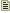# `phys_ragdollconstraint`

`phys_ragdollconstraint` is a point entity available in all  games. It is a constraint that fixes the position of two entities, relative to this constraint's origin. Also allows for limits on the rotation around each axis, in the space of this constraint.

## Keyvalues

X axis min limit `(xmin)` <float>
-180 min and 180 max = no constraint on X axis. Default value is -90
X axis max limit `(xmax)` <float>
-180 min and 180 max = no constraint on X axis. Default value is 90
Y axis min limit `(ymin)` <float>
-180 min and 180 max = no constraint on Y axis. Default value is 0
Y axis max limit `(ymax)` <float>
-180 min and 180 max = no constraint on Y axis. Default value is 0
Z axis min limit `(zmin)` <float>
-180 min and 180 max = no constraint on Z axis. Default value is 0
Z axis max limit `(zmax)` <float>
-180 min and 180 max = no constraint on Z axis. Default value is 0
X axis friction `(xfriction)` <float>
Y axis friction `(yfriction)` <float>
Z axis friction `(zfriction)` <float>

TwoObjectPhysics:
Entity 1 `(attach1)` <targetname>
Entity 2 `(attach2)` <targetname>
The entities to constrain. Leave one or the other field blank to constrain to the world.Note:Only one entity will be constrained, even if several share the given targetname.
Constraint System Manager `(constraintsystem)` <targetname>
A `phys_constraintsystem` that this constraint should be a part of. This avoids the "jiggling" caused by constraints applied to the same set of entities fighting with each other.
Force Limit to Break `(forcelimit)` <float>
Impact force required to break the constraint, in pounds. 0 means infinite. A way of calculating this is to set it to the weight of an object that would break the constraint if it were resting on its objects.
Torque Limit to Break `(torquelimit)` <float>
Torque required to break the constraint, in pounds*inches. 0 means infinite. A way of calculating this is to multiply any reference mass by the resting distance (from the center of mass of the object) needed to break the constraint.
Play Sound on Break `(breaksound)` <string>
A sound played when the constraint is broken.
Follow teleport distance `(teleportfollowdistance)` <float>
If one constrained object teleports more than this many units away, the other will teleport with it.

Targetname:
Name `(targetname)` <string>
The targetname that other entities refer to this entity by.

## Flags

• 1 : No Collision until break
• 2 : Only limit rotation (free movement)
•  [`1`] : No Collision until break
•  [`4`] : Start inactive
•  [`8`] : Change mass to keep stable attachment to world
•  [`16`] : Do not connect entities until turned on

## Inputs

TwoObjectPhysics:
`Break`
Force the constraint to break.
`TurnOn`
Enable the constraint; do this if the constrained objects don't exist when it spawns. Note that broken constraints cannot be turned back on as they have been deleted.
`TurnOff`
Disable the constraint.

## Outputs

TwoObjectPhysics:
`OnBreak`
Fired when the constraint breaks.